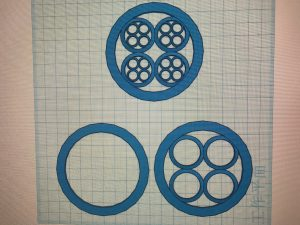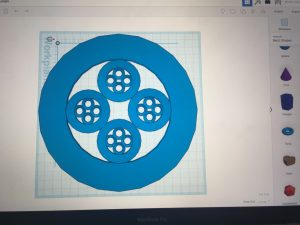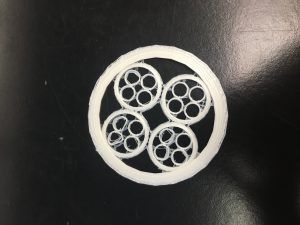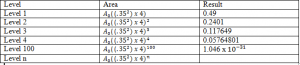# Fruit Loops Fractal

### Group Members

Megan Shanian (Designer)

Jiahao Guo (Math Consultant)

Cat Falvey (Writer)

We created a circular fractal for this assignment. None of us had ever seen anything like this, so we thought it would be perfect to experiment with and present to the class.

This design is a fractal because it is simply a repeating pattern of circles inside of each other on an identical scale. Mathematically speaking, Jiahao used a scale of 0.35 to decrease the size of each consecutive set of circles. The largest circle has a diameter of 80 units, the medium circles have a diameter of 28 units, and the smallest circles share a diameter of 9.80 units. Then, Jiahao used a ruler to ensure that the circles were all parallel and lined up correctly.[The intital design sketches]

During the initial designing period, Megan created the base design for this fractal using the “torus” shape in Tinkercad. Instead of using multiple elements, she stuck to using one over and over again. By cropping the shapes and moving them around the workplane accordingly, she was able to create a fully functional fractal with the same “ring-like” shape.

In order to make sure the same sized circles were all completely identical, Megan used the copy function on Tinkercad for each size and just pasted it however many times corresponded with the number of circles we needed for that respective step.Initial Calculations:

At level 0, the diameter is 100u and the width of the circle is 7u. The equation for the area of level 0 is: A = π(50²), which equals 7853.98u. The equation for the area of the outer circle is: A =π(43²), which equals 5808.81mm. To find the total area we subtracted the area of the outer circle from the original area. The answer was 250.53mm. We multiplied this by .35², which came out to .1125. 0.35 was our original scale for the design. We multiplied by .1125u to get 28.19mm as the area for level 2.#### 1 Comment on Fruit Loops Fractal

1. For your calculations, you need to show what is happening for levels 1, 2, 3, 4, … until the pattern is clear, then level 100, level “n”, and as the levels go to infinity. Also, what is “u”? See if you can organize your work better so that your mathematics is clearer.